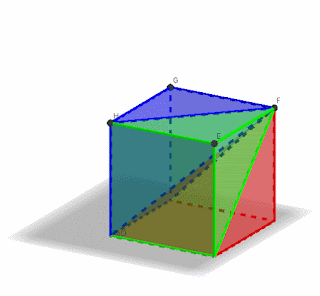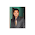# BP Dhakal

## Sunday, December 8, 2019

A pyramid is a polyhedron with one base that is any polygon . Its other faces are triangles. The volume V of a pyramid is one-third the area of the base B times the height h.
i.e., V=1/3 * B* h where B is the area of the base and h is the height of the pyramid.In the given animation above
﻿ ﻿ Volume of Cube = Volume of 3 pyramids or Volume of Pyramid= 1/3 x Volume of Cube
or Volume of Pyramid= 1/3 x B *h
where B is the area of the base and h is the height of the pyramid. (Cube र Pyramid को base area र उचाई एउटै छ )

## Dynamic Worksheet in GeoGebra

1.सर यसको आधार वृत्तकार हुन सक्छ?
अथवा सोली पिरामिड हो?

1.सोलि बृताकार पिरमिड हो ।

2.2.3.